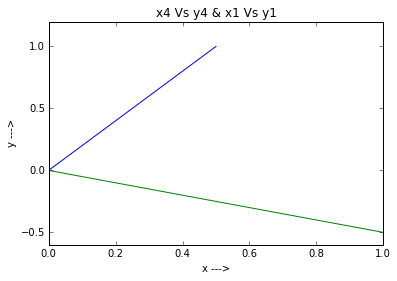# Chapter 14 : Optimum signal detection¶

## Page 631 Prob 14.1¶

In :
from numpy import arange
%matplotlib inline
from matplotlib.pyplot import plot,show,title,xlabel,ylabel

# the co-ordinates of the vectors are
# s1(1,-0.5),s2=(-0.5,1),s3=(0,-1),s4=(0.5,1)
x4=arange(0,0.6,0.1)
y4=[]
for x in x4:y4.append(2*x)

plot(x4,y4) # blue line
x1=arange(0,1.1,0.1)
y1=[]
for x in x1:y1.append(-0.5*x)
plot(x1,y1) #green line
title("x4 Vs y4 & x1 Vs y1")
xlabel("x --->")
ylabel("y --->")
show()## Page no. 650 problem no. 14.2¶

In :
from math import sqrt,log

# the two symbols to be transmitted are m1 and m2,the probabilities of which are not equal
# To design the optimum receiver we need to decide the threshold say d
# N be the given noise  PSD,E the energy of the signal, assume N =1, E=1.5
Pm1=input("probability of symbol m1 is ")
Pm2=input("probability of symbol m2 is")
#d is calculated as follows
N=1#
E=1.5#
d=(N/(4*sqrt(E)))*log(Pm2/Pm1)#
print "the threshold is= %0.2f "%d

probability of symbol m1 is 0.168
probability of symbol m2 is0.245
the threshold is= 0.08


## Page no 665 Problem 14.7¶

In :
# we know k1P(m1)=k2P(m2), where k1 and k2 are the distances of the signals s1 and s2 resp.,hence k1+k2=d
Pm1=input("probability of symbol m1 is")
Pm2=input("probability of symbol m2 is")
#assume d=1
d=1#
E1=(((Pm1)*((d**2)/2.))+((Pm2)*((d**2)/2)))#
print "mean energy of the first signal is %f units"%E1
E2=Pm1*Pm2*(d**2)#
print "mean energy of the second signal is %f units"%E2
if(E1==E2):
print "signals are equiprobable"#


probability of symbol m1 is0.25
probability of symbol m2 is0.35
mean energy of the first signal is 0.125000 units
mean energy of the second signal is 0.087500 units